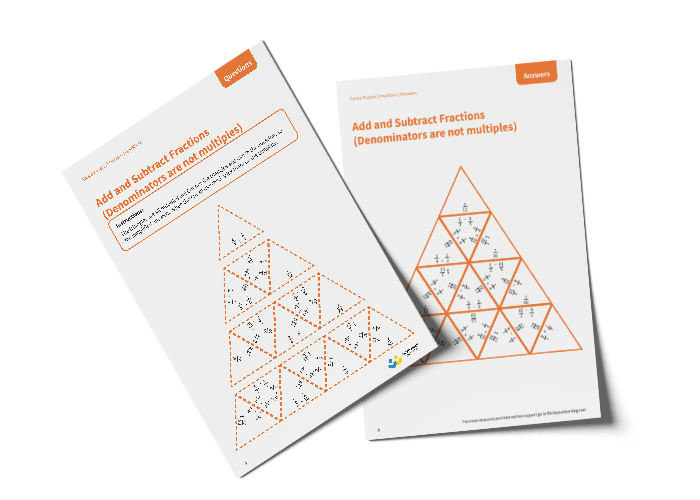# Tarsia Puzzle Add and Subtract Fractions (Denominators are not Multiples) (Year 6)

The triangles in the tarsia puzzle have all been mixed up. In this adding and subtracting fractions (where the denominators are not multiples) tarsia puzzle, pupils will need to match the addition or subtraction question to the correct answer to create one large triangle.

This Year 6 add and subtract fractions (denominators are not multiples) tarsia puzzle worksheet contains:

• One question sheet
• One template sheet

Year 6

Fractions